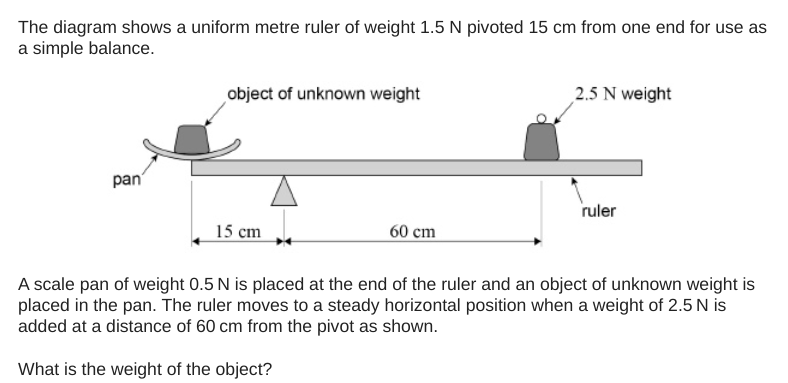## Just a moment . . .

Many years ago, I was taught this compact and intuitive convention to show turning moments. I think it should be more widely known, as it not only is concise and powerful, but also meets the criterion of being an effective form of dual coding which is helpful for both GCSE and A-level Physics students.

Let’s look at an example question.Let’s start by ‘annotating the hell’ out of the diagram.

We could take moments around any of the marked points A-E on the diagram. However, we’re going to take moments around B as it enables us to ignore the upward reaction force acting on the rule at B. (This force is not shown on the diagram.)

To indicate that we’re going to be considering the sum of the clockwise moments about point B, we use this intuitive notation:

If we consider the sum of anticlockwise moments about point B, we use this:

We lay out our calculations of the total clockwise and anticlockwise moments about B as follows.

We show that we are going to apply the Principle of Moments (the sum of clockwise moments is equal to the sum of anticlockwise moments for an object in equilibrium) like this:

The rest, as they say, is not history but algebra:

I hope you find this ‘momentary’ convention useful(!)